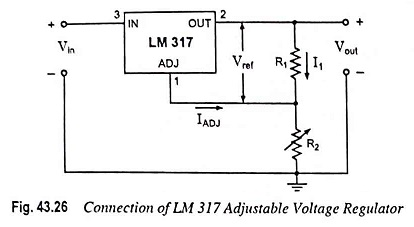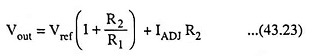Sometimes variable voltage is required and under such a situation adjustable voltage regulators is used. A number of IC regulators, like LM317, LM338, and LM350, are adjustable regulators. They have maximum load currents from 0.10 to 1.5 A.

For example, the LM 317 is a three-terminal positive voltage regulator, which can be operated with the output voltage regulated at any setting over the range of 1.2 V to 37 V. Figure 43.26 illustrates how the regulated output voltage of an LM 317 can be set.Resistors R1 and R2 set the output to any desired voltage over the adjustment range (1.2 to 37 V). The output voltage desired can be determined using equationwhere Vref = 1.25 V, the reference voltage between the output and adjustment terminals.

However, the current IADJ is very small (100 μA) and constant.

So the voltage drop across R2 due to IADJ is also very small and can be neglected so that Eq. (43.23) can be written asThe current set resistor R1 is usually 240Ω, and to achieve good load regulation it should be tied directly to the output of the regulator rather than near the load.

The load regulation is 0.1 per cent while the line regulation is 0.01 per cent per volt. This means that the output voltage varies only 0.01 per cent for each volt of input voltage. The ripple rejection is 80 dB, equivalent to 10,000.

The LM 337 series of adjustable voltage regulators is a complement to the LM317 series devices. The negative adjustable voltage regulators are available in the same voltage and current options as the LM317 devices.

Tables 43.4 and 43.5 show the different grades of the positive and negative regulators in series 317 and 337 respectively.

Scroll to Top